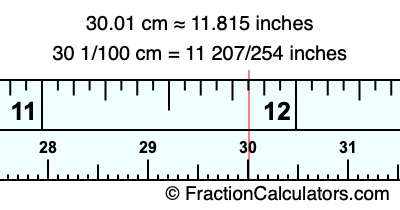30.01 cm in inchesHere is how to convert 30.01 cm to inches. We will give you the fractional answer, the decimal answer, and illustrate the answer on our tape measure.

One centimeter is equal to 50/127 inches, and 30.01 as a fraction is 30 1/100. Therefore, to get the fractional answer to 30.01 cm in inches, we multiply 30 1/100 by 50/127 and simplify if necessary.

cm × 50/127 = inches
30 1/100 × 50/127 = inches
30 1/100 × 50/127 = 11 207/254
30 1/100 cm = 11 207/254 inches
30.01 cm = 11 207/254 inches

As promised above, we also have the answer to 30.01 cm in inches in decimal form. Although the fractional answer above is exact, the decimal answer may be rounded if necessary:

11 207/254 ≈ 11.8149606299213
11 207/254 ≈ 11.815
30.01 cm ≈ 11.815 inches

Our image below shows 30.01 cm on a tape measure. The top row of the tape measure is inches, and the bottom row is centimeters.As you can see, we drew a red line where 30.01 cm and 11.815 inches meet on the measuring tape.

Centimeters to Inches Calculator
Here you can convert another length in centimeters to inches.

Convert  cm to inches.

What is 30.02 cm in inches?
Here is another inches to centimeters calculation we did for you.